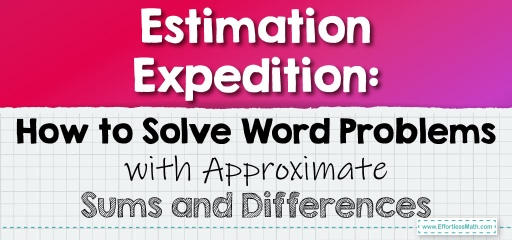# Estimation Expedition: How to Solve Word Problems with Approximate Sums and Differences

In real-life scenarios, we often don't need exact numbers but rather a good estimate. Estimating sums and differences in word problems can help us make quick decisions, plan better, or even check if our detailed calculations are in the right ballpark. Let's explore how to tackle word problems using estimation.## Estimating Sums and Differences in Word Problems

### Example 1:

Lucy went to a bookstore and bought books worth $$4.58$$ dollars and $$5.67$$ dollars. If she gave a $$10$$ dollar bill to the cashier, approximately how much change should she get back?

Estimation Process:

1. Round $$4.58$$ to $$5$$.

2. Round $$5.67$$ to $$6$$.

3. Estimate the total cost: $$5 + 6 = 11$$.

4. Estimate the change from $$10$$ dollars: $$10 – 11 = -1$$.

Lucy would need to add approximately $$1$$ dollar more.

The Absolute Best Book for 5th Grade Students

### Example 2:

John has $$3.42$$ liters of juice and drinks $$1.78$$ liters during lunch. About how much juice does he have left?

Estimation Process:

1. Round $$3.42$$ to $$3$$.

2. Round $$1.78$$ to $$2$$.

3. Estimate the remaining juice: $$3 – 2 = 1$$.

John has approximately $$1$$ liter of juice left.

Estimation is a powerful tool, especially in word problems. It allows for quick calculations and provides a general idea of the outcome. While it’s essential to know how to compute exact values, being able to estimate effectively is a valuable skill in both academic and real-world situations. Practice regularly to hone this skill!

### Practice Questions:

1. Emma bought candies worth $$2.34$$ dollars and $$1.89$$ dollars. If she paid with a $$5$$ dollar bill, approximately how much change should she receive?

2. A tank has $$7.65$$ liters of water, and $$3.49$$ liters are used to water plants. About how much water remains in the tank?

3. Mike traveled $$4.89$$ miles to school and $$5.12$$ miles to the library. Approximately how many miles did he travel in total?

4. A bakery sold $$6.58$$ dollars worth of pastries in the morning and $$7.44$$ dollars in the afternoon. About how much did they make for the day?

5. Lisa read $$3.29$$ hours on Monday and $$4.76$$ hours on Tuesday. Approximately how many hours did she read in total?

A Perfect Book for Grade 5 Math Word Problems!

1. $$1$$ dollar.

2. $$4$$ liters.

3. $$10$$ miles.

4. $$14$$ dollars.

5. $$8$$ hours.

The Best Math Books for Elementary Students

### What people say about "Estimation Expedition: How to Solve Word Problems with Approximate Sums and Differences - Effortless Math: We Help Students Learn to LOVE Mathematics"?

No one replied yet.

X
51% OFF

Limited time only!

Save Over 51%

SAVE $15 It was$29.99 now it is \$14.99